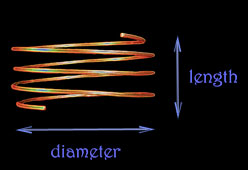## Primary coil turns calculatorCalculates the possible number of turns of wire for the primary coil. The coil used here for this calculation is solenoid shaped.

Input required for this calculation includes these properties of the primary coil:

•  mass (of the copper wire),
•  diameter,
•  and wire gauge.

( units are in:   kg, cm, AWG,   respectively )

The length of the coil (ℓ), in centimeters, is only required later to calculate the coil's inductance.

The mass for this coil should be taken as equal to the mass of the secondary and extra coils. That is, the mass for the primary should be:

M primary   =   M secondary   +   M extra

This tool allows us to try different diameters, lengths, and gauges for the primary, and then it solves for the number of turns the coil would need.

( For example, increasing the wire thickness (smaller gauges) reduces the number of turns. )

mass of copper coil:      kg

diameter:       cm

length:       cm

 wire gauge: 14 13 12 11 10 AWG
submit

 The total number of turns for the coil:         N =  0.0000000000  turns

 mass   (M primary): 0.00000  kg coil diameter  (d) : 0.00  m coil length  (ℓ) : 0.000  m wire gauge   : AWG wire radius (inches)  : 1.00000  in wire radius (meters)  : 1.00000  m wire length (inches)  : 0.00000  in wire length (meters)  : 0.00000  m L   : 0.000000000000   μH

density of copper (ρ)  =  8940 kg / m3

The number of turns (N) is computed from:

 M primary N   = π 2  ρ  d  r 2

where,

•  N   = the number of turns of the coil
•  M primary   = the mass of the coil (kilograms)
•  ρ   = the density of copper (kg / m3)
•  d   = diameter of the coil (meters)
•  r   = radius of the wire (meters)

The above is derived from:

total length of the wire for the coil :    ℓ = N C

( length of the wire = turns, times, circumference of the coil )

and,

total length of the wire for the coil :   ℓ = M / ( ρ A )

( length of the wire = mass of the wire, divided by:
density of copper times
the cross-sectional area of the wire )

The inductance, in μH,  is estimated by the following, with the length and diameter measured in inches. **

Inductance ( μH )

 d 2  N 2 L    = 18 d  +  40 ℓ

** The ARRL Handbook for Radio Communications 2011
section 2.8 Inductance and Inductors

 radiant arcs tesla coils self-oscillating voltage demo audio tests techstars application violet ray documents tools copper wire length calculator capacitance calculator primary coil turns calculator home